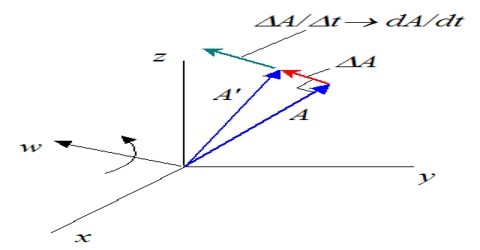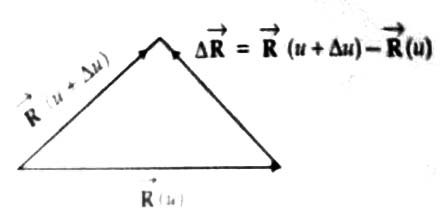# Differentiation of a Vector

Differentiation of a Vector

Let V (u) be a vector that depends on unit scalar operator u. In mathematical language V is a function of u. Then we can write,

∆R/∆u = [∆R(u+∆u) – R (u)] / ∆u

here ∆u, means a small increases of u.∆R/∆u is the rate of change of R with respect to u. When ∆u tends to zero,

lim∆u→0 ∆R/∆u = lim∆u→0 [∆R(u+∆u) – R (u)] / ∆u

lim∆u→0 ∆R/∆u can be written as dR/du.

So, lim∆u→0 ∆R/∆u = dR/du = lim∆u→0 = [∆R(u+∆u) – R (u)] / ∆u

dR/du; means the rate of change of R with respect to u for exceedingly small change of u.

dR/du; called the differential coefficient of R with respect to u.

Process of determining dR/du is called differentiation.